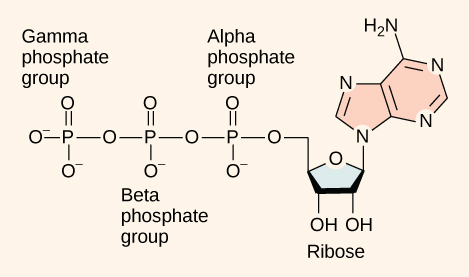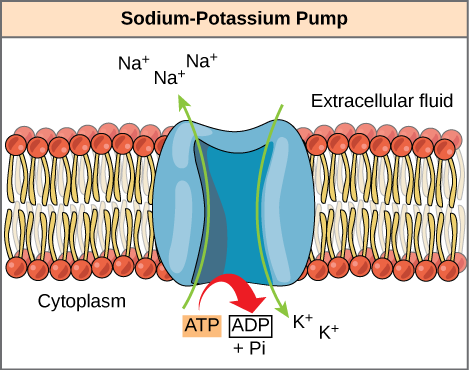Metabolism

### Learning Objectives

By the end of this section, you will be able to do the following:

• Explain ATP’s role as the cellular energy currency
• Describe how energy releases through ATP hydrolysis

Even exergonic, energy-releasing reactions require a small amount of activation energy in order to proceed. However, consider endergonic reactions, which require much more energy input, because their products have more free energy than their reactants. Within the cell, from where does energy to power such reactions come? The answer lies with an energy-supplying molecule scientists call adenosine triphosphate, or ATP. This is a small, relatively simple molecule ((Figure)), but within some of its bonds, it contains the potential for a quick burst of energy that can be harnessed to perform cellular work. Think of this molecule as the cells’ primary energy currency in much the same way that money is the currency that people exchange for things they need. ATP powers the majority of energy-requiring cellular reactions.

ATP is the cell’s primary energy currency. It has an adenosine backbone with three phosphate groups attached.As its name suggests, adenosine triphosphate is comprised of adenosine bound to three phosphate groups ((Figure)). Adenosine is a nucleoside consisting of the nitrogenous base adenine and a five-carbon sugar, ribose. The three phosphate groups, in order of closest to furthest from the ribose sugar, are alpha, beta, and gamma. Together, these chemical groups constitute an energy powerhouse. However, not all bonds within this molecule exist in a particularly high-energy state. Both bonds that link the phosphates are equally high-energy bonds (phosphoanhydride bonds) that, when broken, release sufficient energy to power a variety of cellular reactions and processes. These high-energy bonds are the bonds between the second and third (or beta and gamma) phosphate groups and between the first and second phosphate groups. These bonds are “high-energy” because the products of such bond breaking—adenosine diphosphate (ADP) and one inorganic phosphate group (Pi)—have considerably lower free energy than the reactants: ATP and a water molecule. Because this reaction takes place using a water molecule, it is a hydrolysis reaction. In other words, ATP hydrolyzes into ADP in the following reaction:

$\text{ATP}+{\text{H}}_{\text{2}}\text{O}\to \text{ADP}+{\text{P}}_{\text{i}}+\text{free energy}$

Like most chemical reactions, ATP to ADP hydrolysis is reversible. The reverse reaction regenerates ATP from ADP + Pi. Cells rely on ATP regeneration just as people rely on regenerating spent money through some sort of income. Since ATP hydrolysis releases energy, ATP regeneration must require an input of free energy. This equation expresses ATP formation:

$\text{ADP}+{\text{P}}_{\text{i}}+\text{free energy}\to \text{ATP}+{\text{H}}_{\text{2}}\text{O}$

Two prominent questions remain with regard to using ATP as an energy source. Exactly how much free energy releases with ATP hydrolysis, and how does that free energy do cellular work? The calculated ∆G for the hydrolysis of one ATP mole into ADP and Pi is −7.3 kcal/mole (−30.5 kJ/mol). Since this calculation is true under standard conditions, one would expect a different value exists under cellular conditions. In fact, the ∆G for one ATP mole’s hydrolysis in a living cell is almost double the value at standard conditions: –14 kcal/mol (−57 kJ/mol).

ATP is a highly unstable molecule. Unless quickly used to perform work, ATP spontaneously dissociates into ADP + Pi, and the free energy released during this process is lost as heat. The second question we posed above discusses how ATP hydrolysis energy release performs work inside the cell. This depends on a strategy scientists call energy coupling. Cells couple the ATP hydrolysis’ exergonic reaction allowing them to proceed. One example of energy coupling using ATP involves a transmembrane ion pump that is extremely important for cellular function. This sodium-potassium pump (Na+/K+ pump) drives sodium out of the cell and potassium into the cell ((Figure)). A large percentage of a cell’s ATP powers this pump, because cellular processes bring considerable sodium into the cell and potassium out of it. The pump works constantly to stabilize cellular concentrations of sodium and potassium. In order for the pump to turn one cycle (exporting three Na+ ions and importing two K+ ions), one ATP molecule must hydrolyze. When ATP hydrolyzes, its gamma phosphate does not simply float away, but it actually transfers onto the pump protein. Scientists call this process of a phosphate group binding to a molecule phosphorylation. As with most ATP hydrolysis cases, a phosphate from ATP transfers onto another molecule. In a phosphorylated state, the Na+/K+ pump has more free energy and is triggered to undergo a conformational change. This change allows it to release Na+ to the cell’s outside. It then binds extracellular K+, which, through another conformational change, causes the phosphate to detach from the pump. This phosphate release triggers the K+ to release to the cell’s inside. Essentially, the energy released from the ATP hydrolysis couples with the energy required to power the pump and transport Na+ and K+ ions. ATP performs cellular work using this basic form of energy coupling through phosphorylation.

Visual Connection
The sodium-potassium pump is an example of energy coupling. The energy derived from exergonic ATP hydrolysis pumps sodium and potassium ions across the cell membrane.One ATP molecule’s hydrolysis releases 7.3 kcal/mol of energy (∆G = −7.3 kcal/mol of energy). If it takes 2.1 kcal/mol of energy to move one Na+ across the membrane (∆G = +2.1 kcal/mol of energy), how many sodium ions could one ATP molecule’s hydrolysis move?

Often during cellular metabolic reactions, such as nutrient synthesis and breakdown, certain molecules must alter slightly in their conformation to become substrates for the next step in the reaction series. One example is during the very first steps of cellular respiration, when a sugar glucose molecule breaks down in the process of glycolysis. In the first step, ATP is required to phosphorylze glucose, creating a high-energy but unstable intermediate. This phosphorylation reaction powers a conformational change that allows the phosphorylated glucose molecule to convert to the phosphorylated sugar fructose. Fructose is a necessary intermediate for glycolysis to move forward. Here, ATP hydrolysis’ exergonic reaction couples with the endergonic reaction of converting glucose into a phosphorylated intermediate in the pathway. Once again, the energy released by breaking a phosphate bond within ATP was used for phosphorylyzing another molecule, creating an unstable intermediate and powering an important conformational change.

See an interactive animation of the ATP-producing glycolysis process at this site.

### Section Summary

ATP is the primary energy-supplying molecule for living cells. ATP is comprised of a nucleotide, a five-carbon sugar, and three phosphate groups. The bonds that connect the phosphates (phosphoanhydride bonds) have high-energy content. The energy released from ATP hydrolysis into ADP + Pi performs cellular work. Cells use ATP to perform work by coupling ATP hydrolysis’ exergonic reaction with endergonic reactions. ATP donates its phosphate group to another molecule via phosphorylation. The phosphorylated molecule is at a higher-energy state and is less stable than its unphosphorylated form, and this added energy from phosphate allows the molecule to undergo its endergonic reaction.

### Visual Connection Questions

(Figure) One ATP molecule’s hydrolysis releases 7.3 kcal/mol of energy (∆G = −7.3 kcal/mol of energy). If it takes 2.1 kcal/mol of energy to move one Na+ across the membrane (∆G = +2.1 kcal/mol of energy), how many sodium ions could one ATP molecule’s hydrolysis move?

(Figure) Three sodium ions could be moved by the hydrolysis of one ATP molecule. The ∆G of the coupled reaction must be negative. Movement of three sodium ions across the membrane will take 6.3 kcal of energy (2.1 kcal × 3 Na+ ions = 6.3 kcal). Hydrolysis of ATP provides 7.3 kcal of energy, more than enough to power this reaction. Movement of four sodium ions across the membrane, however, would require 8.4 kcal of energy, more than one ATP molecule can provide.

### Review Questions

The energy released by the hydrolysis of ATP is____

1. primarily stored between the alpha and beta phosphates
2. equal to −57 kcal/mol
3. harnessed as heat energy by the cell to perform work
4. providing energy to coupled reactions

D

Which of the following molecules is likely to have the most potential energy?

1. sucrose
2. ATP
3. glucose

A

### Critical Thinking Questions

Do you think that the EA for ATP hydrolysis is relatively low or high? Explain your reasoning.

The activation energy for hydrolysis is very low. Not only is ATP hydrolysis an exergonic process with a large −∆G, but ATP is also a very unstable molecule that rapidly breaks down into ADP + Pi if not utilized quickly. This suggests a very low EA since it hydrolyzes so quickly.

### Glossary

ATP
adenosine triphosphate, the cell’s energy currency
phosphoanhydride bond
bond that connects phosphates in an ATP molecule